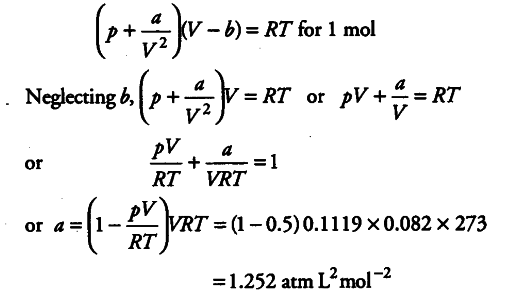# The compression factor (compressibility factor) for one mole of a van

The compression factor (compressibility factor) for one mole of a van der Waals gas at 0°C and 100 atm pressure is found to be 0.5. Assuming that the volume of a gas molecule is negligible, calculate the van der Waals’ constant a.

Z = pV/nRT
i.e., 0.5 = 100 x V / 1 X 0.082 X 273
or V = 0.1119 L